Type
Quiz
Book Title
Fundamentals of Corporate Finance Standard Edition 9th Edition
ISBN 13
978-0073382395

### 978-0073382395 Chapter 16 Questions and Problems 11-22

April 3, 2019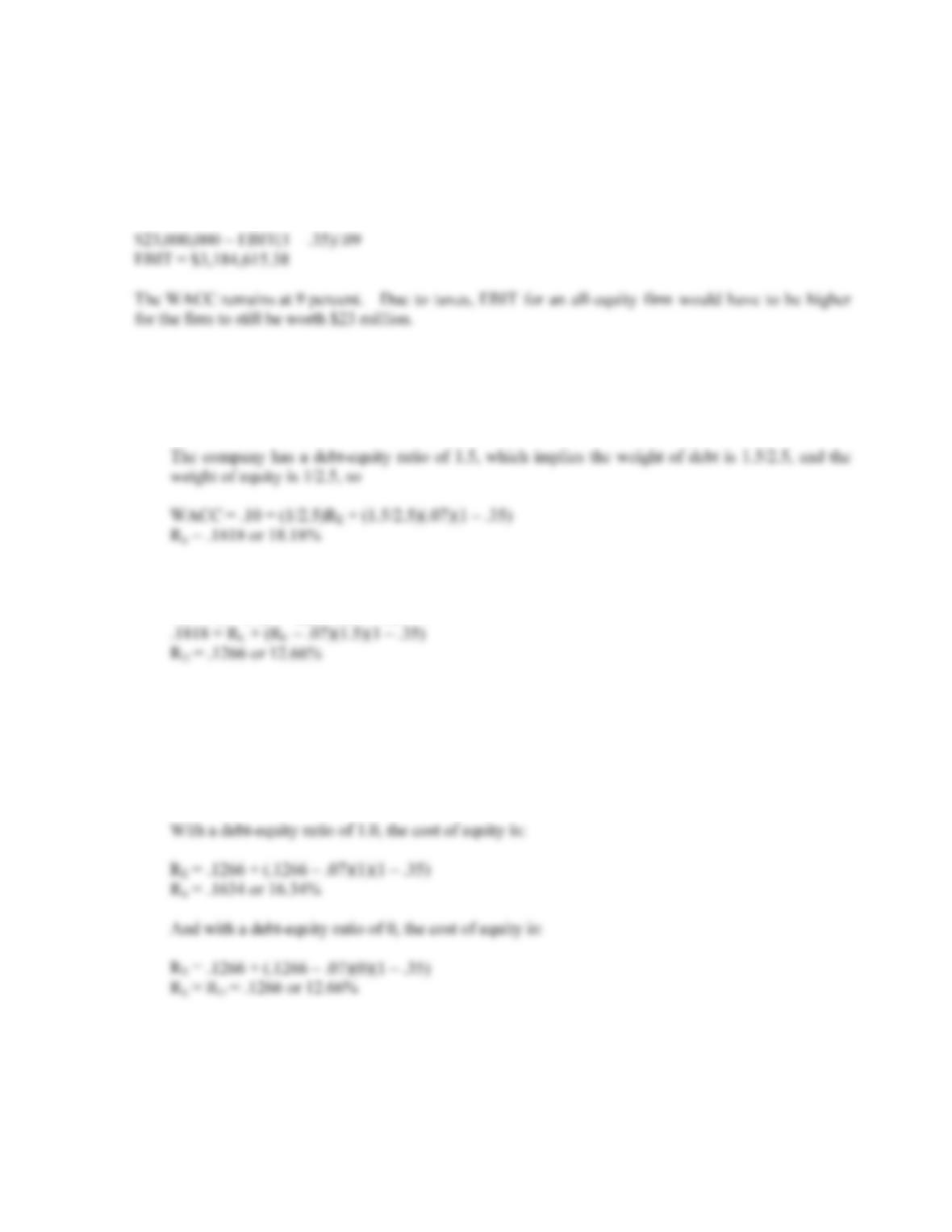B-286 SOLUTIONS
11. If there are corporate taxes, the value of an unlevered firm is:
V
U = EBIT(1 – tC)/RU
Using this relationship, we can find EBIT as:
12. a. With the information provided, we can use the equation for calculating WACC to find the cost of
equity. The equation for WACC is:
WACC = (E/V)RE + (D/V)RD(1 – tC)
b. To find the unlevered cost of equity we need to use M&M Proposition II with taxes, so:
R
E = RU + (RU – RD)(D/E)(1 – tC)
c. To find the cost of equity under different capital structures, we can again use M&M Proposition II
with taxes. With a debt-equity ratio of 2, the cost of equity is:
R
E = RU + (RU – RD)(D/E)(1 – tC)
R
E = .1266 + (.1266 – .07)(2)(1 – .35)
R
E = .2001 or 20.01%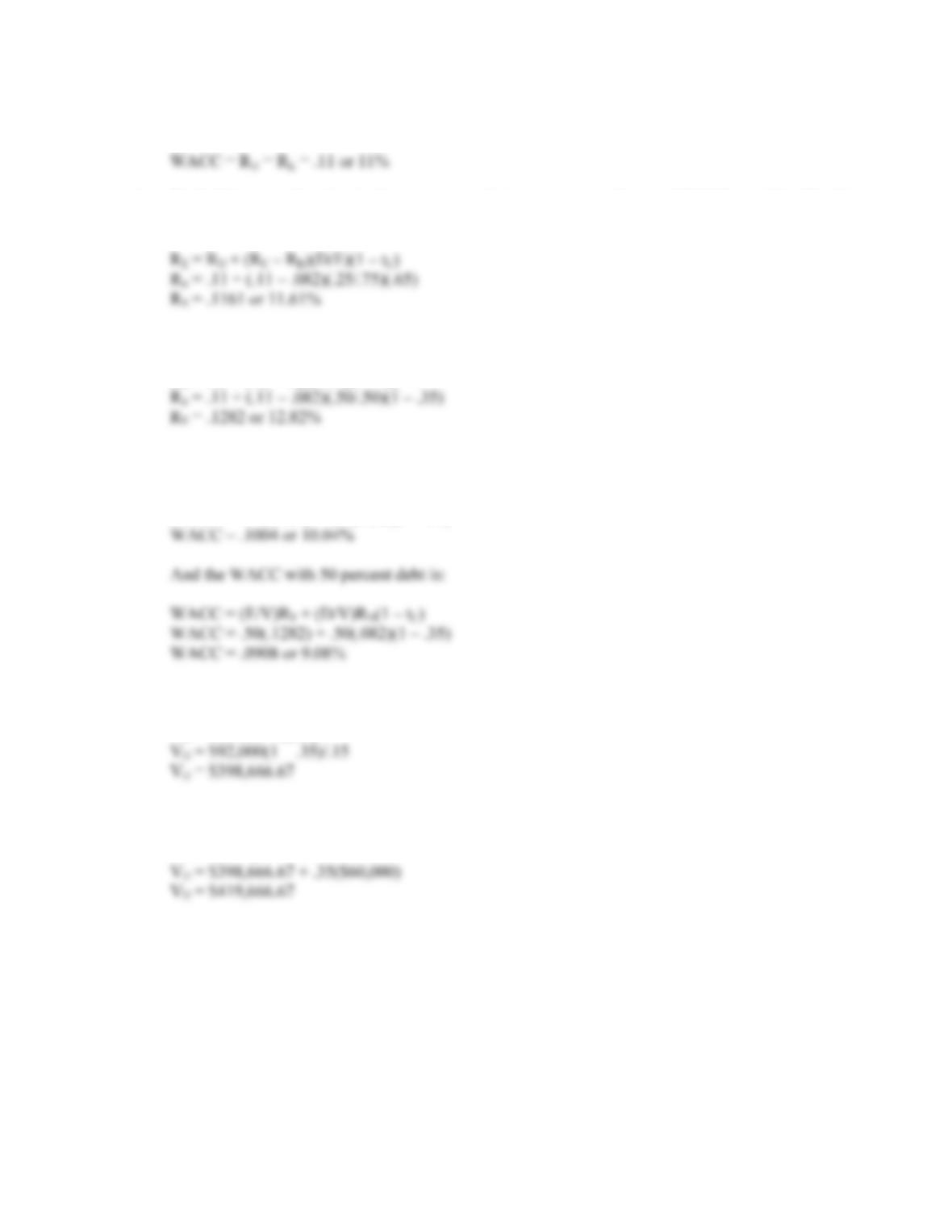CHAPTER 16 B-287
13. a. For an all-equity financed company:
b. To find the cost of equity for the company with leverage we need to use M&M Proposition II with
taxes, so:
c. Using M&M Proposition II with taxes again, we get:
R
E = RU + (RU – RD)(D/E)(1 – tC)
d. The WACC with 25 percent debt is:
WACC = (E/V)RE + (D/V)RD(1 – tC)
WACC = .75(.1161) + .25(.082)(1 – .35)
14. a. The value of the unlevered firm is:
V
U = EBIT(1 – tC)/RU
b. The value of the levered firm is:
V
U = VU + tCD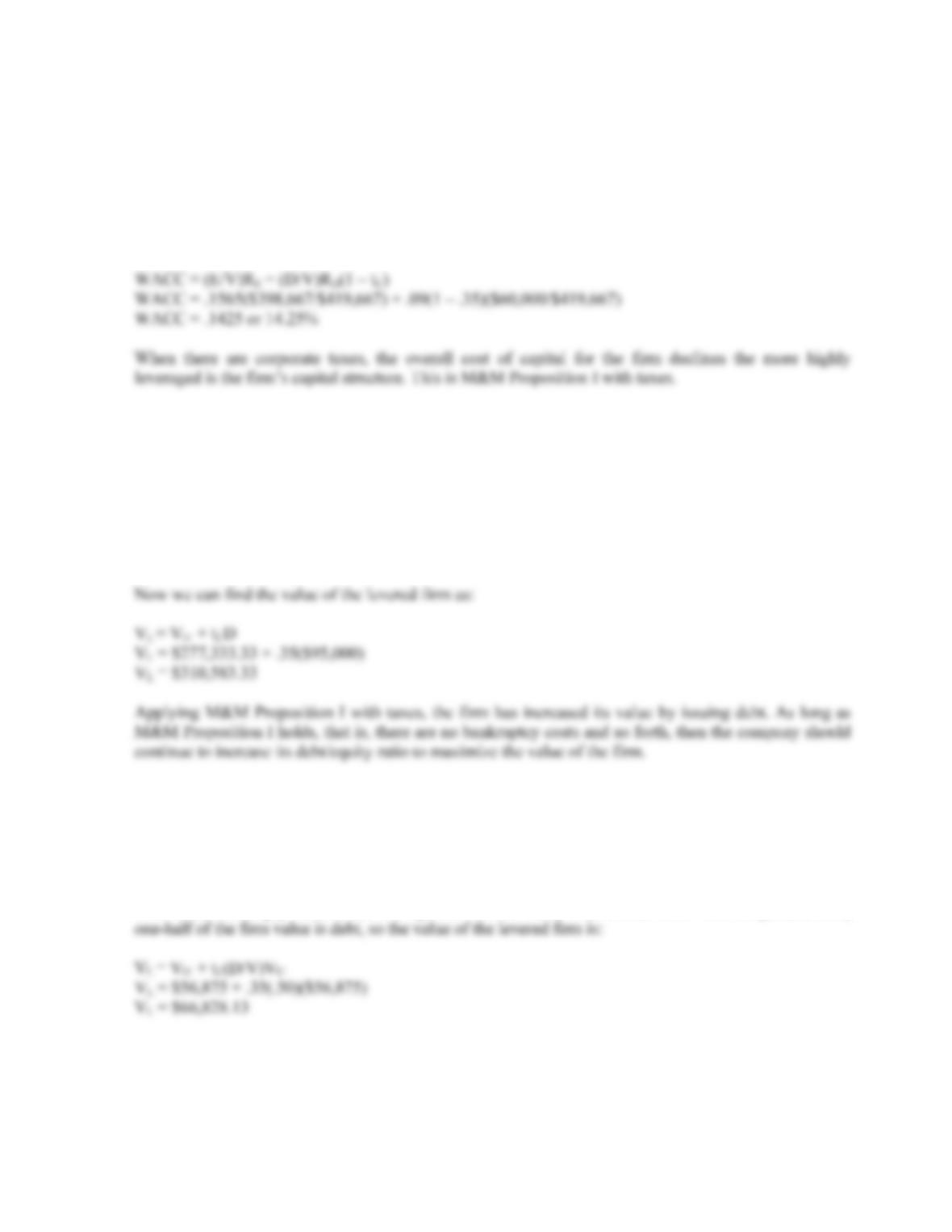B-288 SOLUTIONS
15. We can find the cost of equity using M&M Proposition II with taxes. Doing so, we find:
R
E = RU + (RU – RD)(D/E)(1 – t)
R
E = .15 + (.15 – .09)(\$60,000/\$398,667)(1 – .35)
R
E = .1565 or 15.65%
Using this cost of equity, the WACC for the firm after recapitalization is:
Intermediate
16. To find the value of the levered firm we first need to find the value of an unlevered firm. So, the value of
the unlevered firm is:
V
U = EBIT(1 – tC)/RU
V
U = (\$64,000)(1 – .35)/.15
V
U = \$277,333.33
17. With no debt, we are finding the value of an unlevered firm, so:
V
U = EBIT(1 – tC)/RU
V
U = \$14,000(1 – .35)/.16
V
U = \$56,875
With debt, we simply need to use the equation for the value of a levered firm. With 50 percent debt,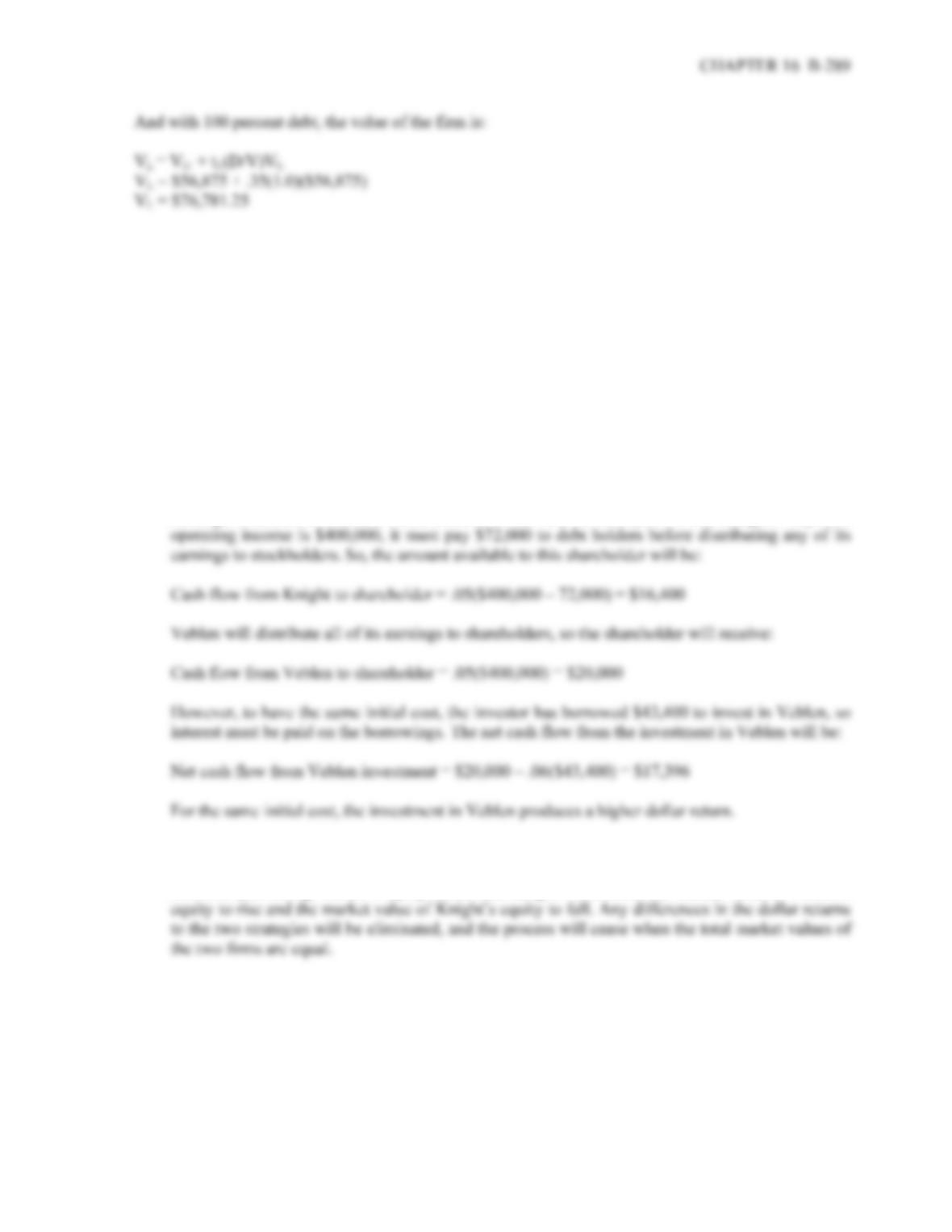18. a. To purchase 5 percent of Knight’s equity, the investor would need:
Knight investment = .05(\$1,632,000) = \$81,600
And to purchase 5 percent of Veblen without borrowing would require:
Veblen investment = .05(\$2,500,000) = \$125,000
In order to compare dollar returns, the initial net cost of both positions should be the same.
Therefore, the investor will need to borrow the difference between the two amounts, or:
Amount to borrow = \$125,000 – 81,600 = \$43,400
An investor who owns 5 percent of Knight’s equity will be entitled to 5 percent of the firm’s
earnings available to common stock holders at the end of each year. While Knight’s expected
b. Both of the two strategies have the same initial cost. Since the dollar return to the investment in
Veblen is higher, all investors will choose to invest in Veblen over Knight. The process of
investors purchasing Veblen’s equity rather than Knight’s will cause the market value of Veblen’s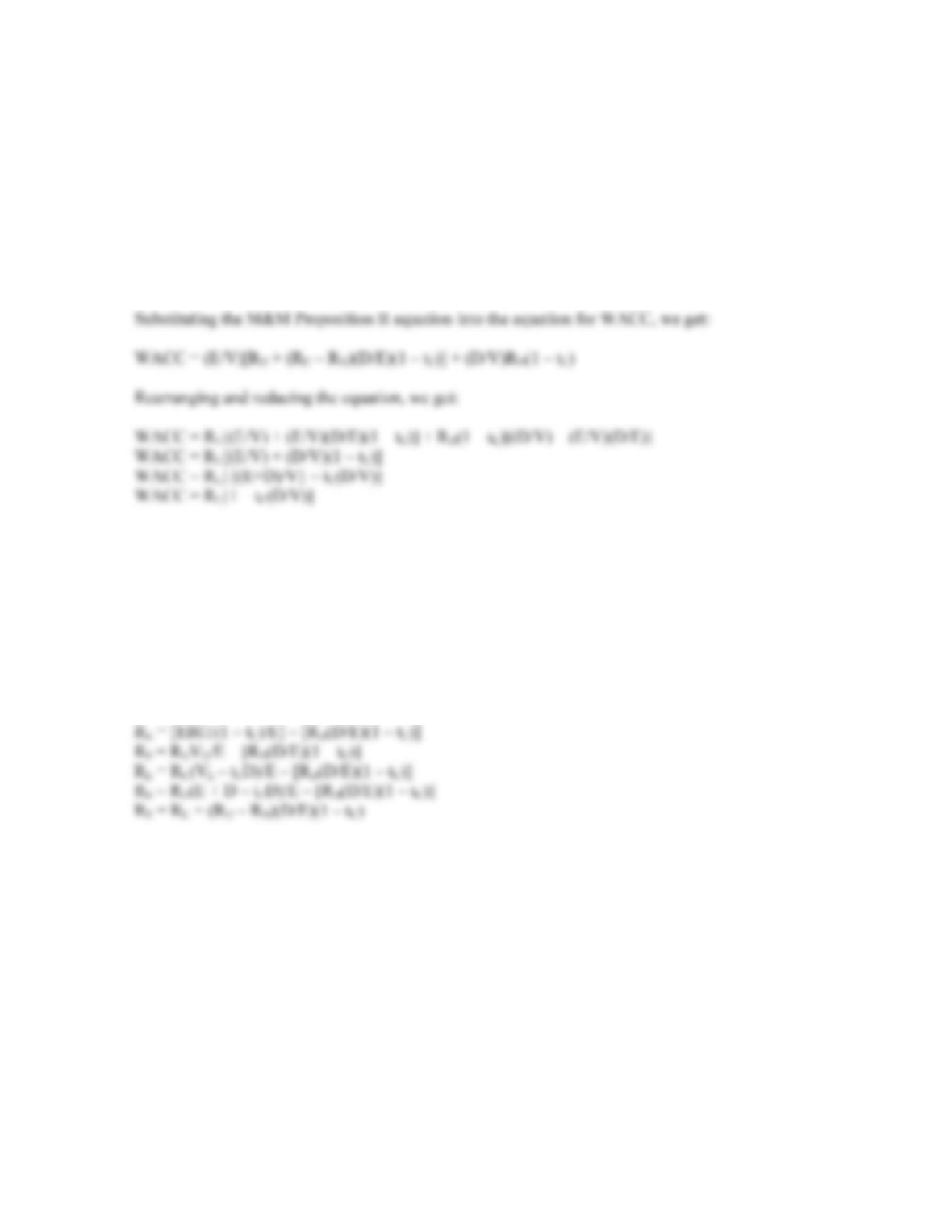B-290 SOLUTIONS
Challenge
19. M&M Proposition II states:
R
E = RU + (RU – RD)(D/E)(1 – tC)
And the equation for WACC is:
WACC = (E/V)RE + (D/V)RD(1 – tC)
20. The return on equity is net income divided by equity. Net income can be expressed as:
NI = (EBIT – RDD)(1 – tC)
So, ROE is:
R
E = (EBIT – RDD)(1 – tC)/E
Now we can rearrange and substitute as follows to arrive at M&M Proposition II with taxes:
R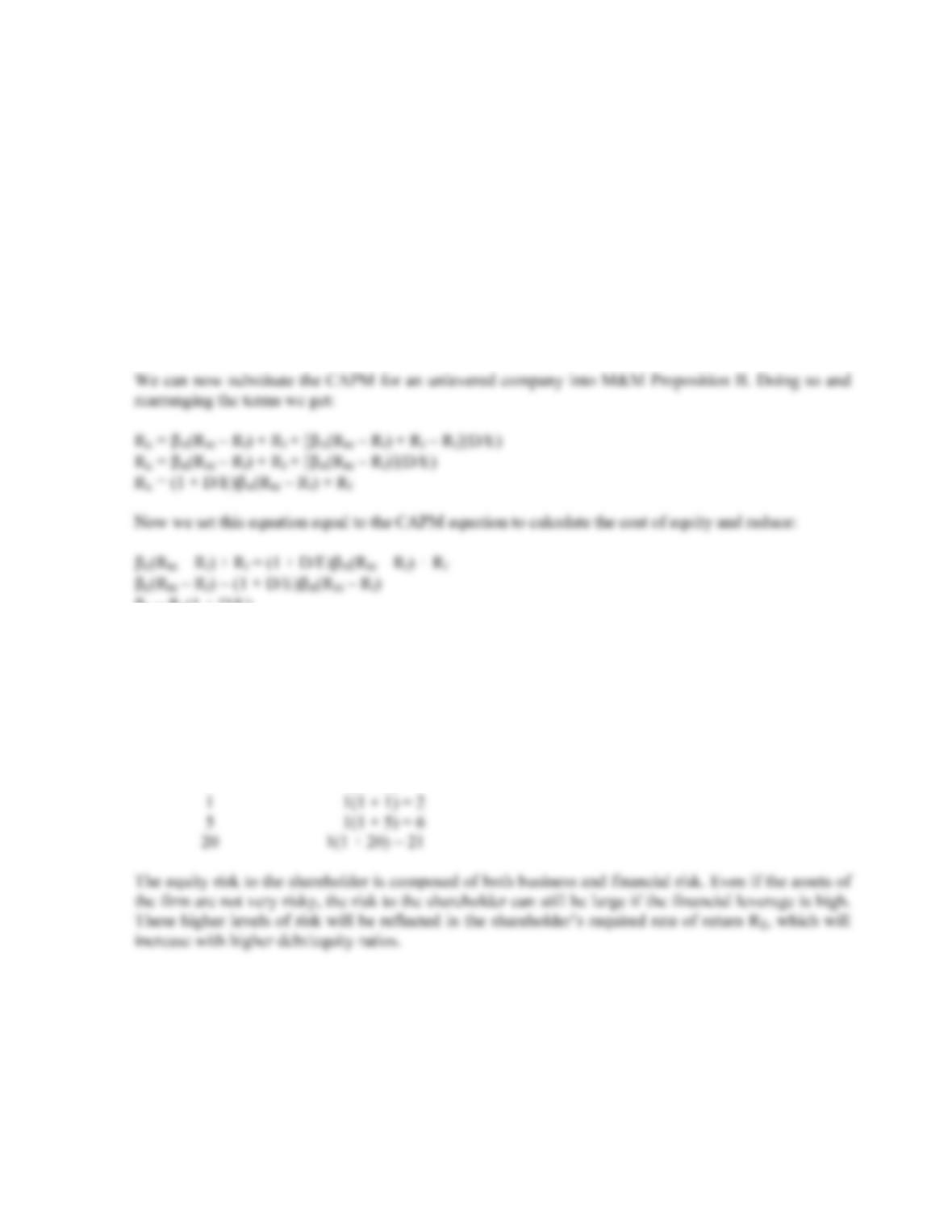CHAPTER 16 B-291
21. M&M Proposition II, with no taxes is:
R
E = RU + (RU – Rf)(D/E)
Note that we use the risk-free rate as the return on debt. This is an important assumption of M&M
Proposition II. The CAPM to calculate the cost of equity is expressed as:
R
E = E(RM – Rf) + Rf
We can rewrite the CAPM to express the return on an unlevered company as:
R
A = A(RM – Rf) + Rf
E = A(1 + D/E)
22. Using the equation we derived in Problem 21:
E = A(1 + D/E)
The equity beta for the respective asset betas is:
Debt-equity ratio Equity beta
0 1(1 + 0) = 1